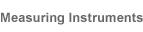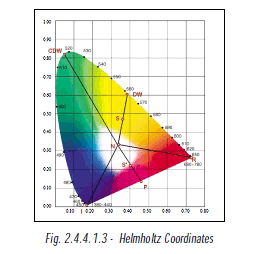## Language of Light

## 2.Concepts

### 2.4 COLORIMETRY

#### 2.4.4.1 TRISTIMULUS COLORIMETRY

##### 2.4.4.1.3 HELMHOLTZ COORDINATESAn alternative set of coordinates in the CIE system, Dominant Wavelength and Purity (also known as Helmholtz coordinates), correlate more closely with the visual aspects of hue and chroma. The dominant wavelength (DW) of a colour is the wavelength of the spectrum colour whose chromaticity is on the same straight line as the sample point (S) and the illuminant point (N) (for light source measurement, the illuminant point is x=0.333 and y=0.333). Purity, also known as excitation purity, is the distance from the illuminant point (N) to the sample point (S), divided by that from the illuminant point (N) to the spectrum locus (DW).
Purity = (N—S) / (N—DW)
The above method is only applicable to spectral colour, that is colour which appears in visible spectrum. When measurement of non-spectral colour, that is colour which does not appear in visible spectrum and is located within the triangle area encompassed by the 3 points N, R and B, is concerned, Complementary Dominant Wavelength (CDW) is used. This is because the interception point P, which is supposed to be the Dominant Wavelength has no corresponding wavelength. The line from N to P is extended backward in order to determine the Complementary Dominant Wavelength (CDW). Purity for non-spectral colour is calculated from:
Purity = (N—S’) / (N—P)
Dominant wavelength and purity are commonly used in LEDs’ colour specification.